# PSAT Math : How to find permutation notation

## Example Questions

### Example Question #1893 : Psat Mathematics

A company assigns employee numbers according to the following scheme:

1) Each number must comprise three letters, followed by five numerals.

2) The first numeral must be either '1' or '2'; there are no other restrictions on the numerals.

3) There cannot be repetition among the three letters.

4) To prevent confusion with the numerals '1' and '0', the letters 'I' and 'O' cannot appear.

Which of the following expressions is equal to the number of possible employee numbers?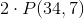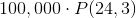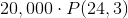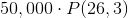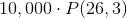Explanation:

The first three characters must be distinct letters, none of which are 'I' or 'O', meaning that three letters will be selected from a set of 24. Also, order will be important, so the number of ways to choose this group of three will be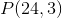.

The letters are followed by either a '1' or a '2', so this makes 2 possibilities.

The next three characters will be numerals, and there will be no restrictions, so the number of ways to choose this group will be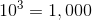.

By the multiplication principle, the number of ways to choose an employee number will be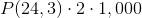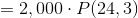### Example Question #11 : Permutation / Combination

A company assigns employee numbers according to the following scheme:

1) Each number must comprise three letters, followed by four numerals, followed by one letter.

2) There are no restrictions on the numerals.

3) There cannot be repetition among the first three letters; however, the final letter can be any letter, even if that letter is among the first three.

Which of the following expressions is equal to the number of possible employee numbers?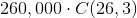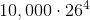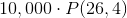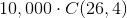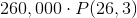Explanation:

The first three characters must be distinct letters, meaning that three letters will be selected from a set of 26. Also, order will be important, so the number of ways to choose this group of three will be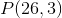.

The next four characters will be numerals, with no restrictions, so the number of ways to choose this group will be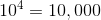The last character can be any of 26 letters.

By the multiplication principle, the number of ways to choose an employee number will be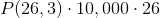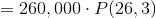### Example Question #12 : Permutation / Combination

A company assigns employee numbers according to the following scheme:

1) Each number must comprise two letters, followed by four numerals, followed by two letters.

2) There are no restrictions on the numerals.

3) There cannot be repetition between the first two letters.

4) There cannot be repetition between the last two letters.

5) A letter appearing as one of the first two letters can appear as one of the last two.

Which of the following expressions is equal to the number of possible employee numbers?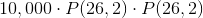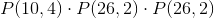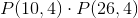Explanation:

The first two characters must be distinct letters, meaning that two letters will be selected from a set of 26. Also, order will be important, so the number of ways to choose this group of two will be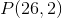.

Similarly, since the last two characters will be chosen according to the same rule, with repetition allowed between the two groups, there will beways to choose them as well.

The next four characters will be numerals, but there will be no restrictions, so the number of ways to choose this group will be.

By the multiplication principle, the number of ways to choose an employee number will be